Kinetic theory of an ideal gas is composed of a large number of tiny invisible particles know as molecules. These molecules are always in state of motion with varying velocities in all possible directions. Molecules traverse straight line path between any two collisions. Size of molecule is infinitely small compared to the average distance traverse by the molecules between any two consecutive collisions. Molecules exert force on each other except when they collide and all of their molecular energy is kinetic.

Intermolecular distance in gas is much larger than that of solids and liquids and the molecules of gas are free to move in entire space free to them.

## Pressure of an ideal Gas

Consider a gas enclosed in a cube of side 1. Take the axes to be parallel to the sides of the cube, as shown in fig.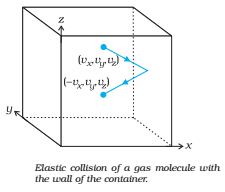1. A molecule with velocity (vx , vy , vz) hits the planar wall parallel to yz-plane of area A (= l2).
2. Since the collision is elastic, the molecule rebounds with the same velocity; its y and z components of velocity do not change in the collision but the x-component reverses sign. So the velocity after collision is
(-vx , vy , vz)
3. The change in momentum of the molecule is: -mvx – (mvx) = -2mvx
By the principle of conservation of momentum, the momentum imparted to the wall in the collision = 2mvx
4. On the average, half of such molecules are moving towards the wall and other half away from the wall.
∴ Number of molecules hitting wall of area A in time ∆t =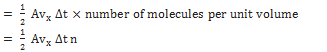5. Total momentum transferred to the wall in time ∆t is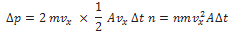6. Force exerted on the wall of area A7. Pressure on the wall =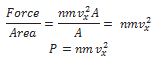As the molecules move with different velocities, so we replace by its average value in the above equation.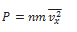1. Again, the gas is isotropic. So the molecular velocities are almost equally distributed in different directions. By symmetry,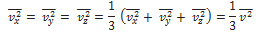Where v2 is the mean square velocity of the molecules:

1.  Hence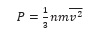1. Density of gas,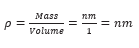Therefore,According to Pascal’s law, a gas transmits pressure equally in all direction. So the equation of point 9 and 10 give the pressure exerted by the gas in any direction.